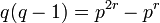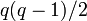# Subgroup structure of projective general linear group of degree two over a finite field

This article gives specific information, namely, subgroup structure, about a family of groups, namely: projective general linear group of degree two.
View subgroup structure of group families | View other specific information about projective general linear group of degree two

## Particular cases

Group$p$$q$ Order of the group Subgroup structure page
symmetric group:S3 2 2 6 subgroup structure of symmetric group:S3
symmetric group:S4 3 3 24 subgroup structure of symmetric group:S4
alternating group:A5 2 4 60 subgroup structure of alternating group:A5
symmetric group:S5 5 5 120 subgroup structure of symmetric group:S5
projective general linear group:PGL(2,7) 7 7 336 subgroup structure of projective general linear group:PGL(2,7)
projective general linear group:PGL(2,9) 3 9 720 subgroup structure of projective general linear group:PGL(2,9)

## Sylow subgroups

We consider the group$PGL(2,q)$ over the field$\mathbb{F}_q$ of$q$ elements.$q$ is a prime power of the form$p^r$ where$p$ is a prime number and$r$ is a positive integer.$p$ is hence also the characteristic of$\mathbb{F}_q$. We call$p$ the characteristic prime.

### Sylow subgroups for the characteristic prime

Item Value
order of$p$-Sylow subgroup$q = p^r$
index of$p$-Sylow subgroup$q^2 - 1 = (q- 1)(q +1) = p^{2r} - 1$
explicit description of one of the$p$-Sylow subgroups image of unitriangular matrix group of degree two:$\{ \begin{pmatrix} 1 & b \\ 0 & 1 \\\end{pmatrix} \mid b \in \mathbb{F}_q \}$
isomorphism class of$p$-Sylow subgroup additive group of$\mathbb{F}_q$, which is an elementary abelian group of order$q = p^r$, i.e., a direct product of$r$ copies of the cyclic group of order$p$
explicit description of$p$-Sylow normalizer image of Borel subgroup of degree two in$GL(2,q)$:$\{ \begin{pmatrix} a & b \\ 0 & c \\\end{pmatrix} \mid a,c \in \mathbb{F}_q^\ast, b \in \mathbb{F}_q \}$
isomorphism class of$p$-Sylow normalizer general affine group of degree one$GA(1,q)$
order of$p$-Sylow normalizer$q(q - 1) = p^{2r} - p^r$$p$-Sylow number (i.e., number of$p$-Sylow subgroups) = index of$p$-Sylow normalizer$q + 1$ (congruent to 1 mod p, as expected from the congruence condition on Sylow numbers)

### Sylow subgroups for other primes: cases and summary

For any prime$\ell$, the$\ell$-Sylow subgroup is nontrivial iff$\ell \mid q^3 - q$. If$\ell \ne p$, then it does not divide$q$, so we get that$\ell \mid q^2 - 1$ which means that either$\ell \mid q - 1$ or$\ell \mid q + 1$. Further, if$\ell \ne 2$, exactly one of these cases can occur. For$\ell = 2$, we make cases based on the residue of$q$ mod 4:

Case on$\ell$ and$q$ Isomorphism type of$\ell$-Sylow subgroup Isomorphism type of$\ell$-Sylow normalizer Order of$\ell$-Sylow normalizer$\ell$-Sylow number = index of$\ell$-Sylow normalizer$\ell$ is an odd prime dividing$q - 1$,$p = 2$ cyclic group dihedral group$2(q - 1)$$q(q + 1)/2$$\ell$ is an odd prime dividing$q - 1$,$p \ne 2$ cyclic group dihedral group$2(q - 1)$$q(q + 1)/2$$\ell$ is an odd prime dividing$q + 1$,$p = 2$ cyclic group dihedral group$2(q + 1)$$q(q - 1)/2$$\ell$ is an odd prime dividing$q + 1$,$p \ne 2$ cyclic group dihedral group$2(q + 1)$$q(q - 1)/2$$\ell = 2$ and$q \equiv 1 \pmod 4$ dihedral group dihedral group largest power of 2 dividing the order = twice the largest power of 2 dividing$q - 1$ largest odd number dividing the order$\ell = 2$ and$q \equiv 3 \pmod 4$ dihedral group dihedral group largest power of 2 dividing the order = twice the largest power of 2 dividing$q + 1$ largest odd number dividing the order